# 2019 - SIGMOD - Uncertainty Annotated Databases - A Lightweight Approach for Approximating Certain Answers

Aug. 2, 2019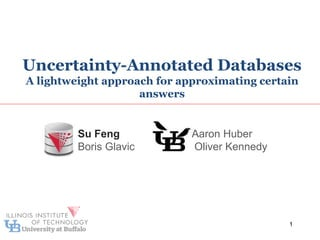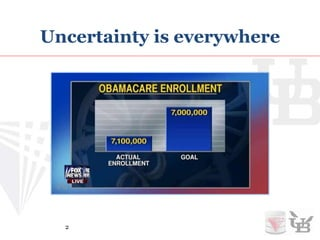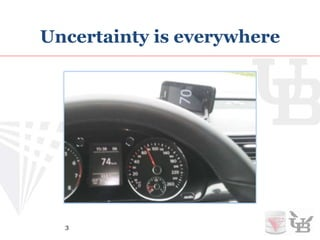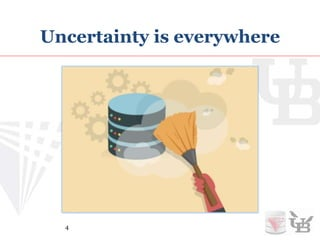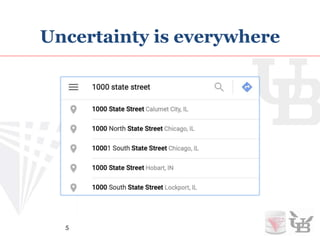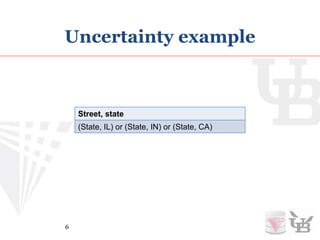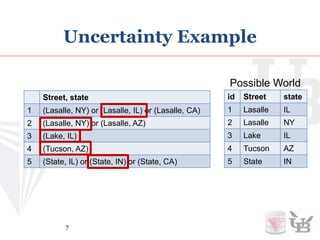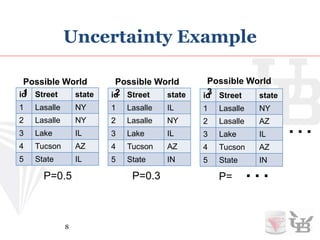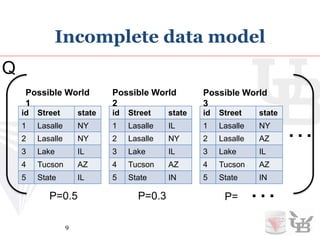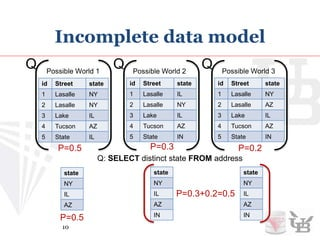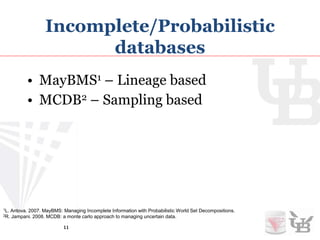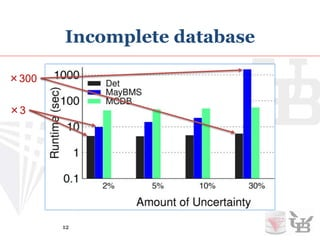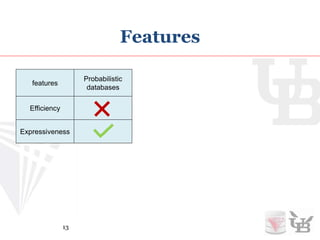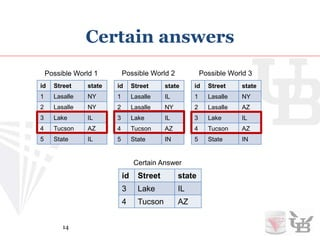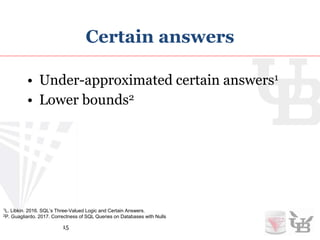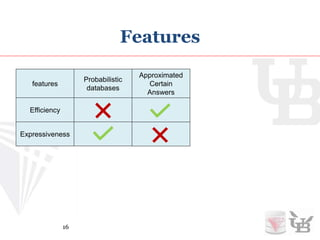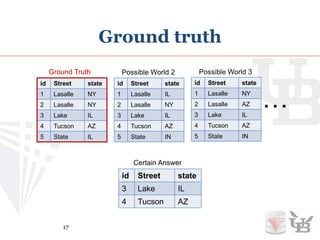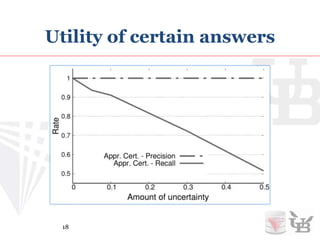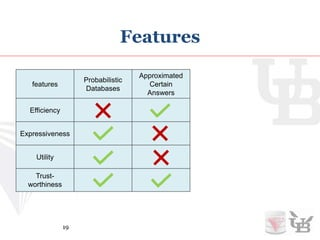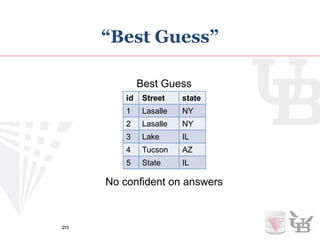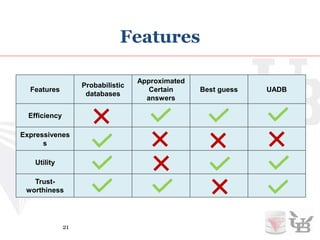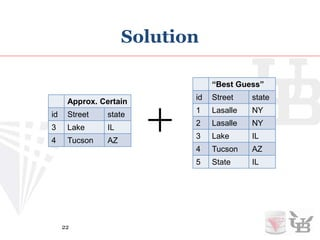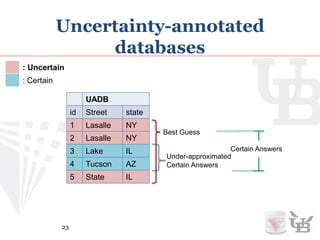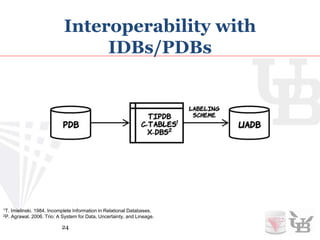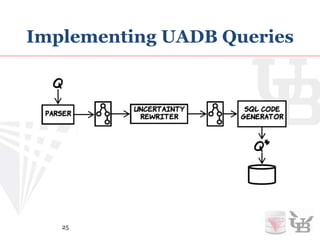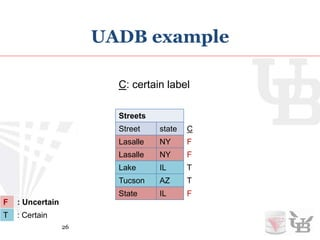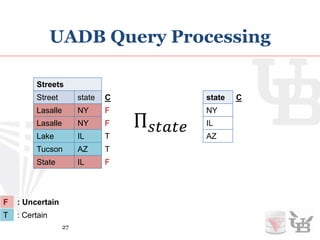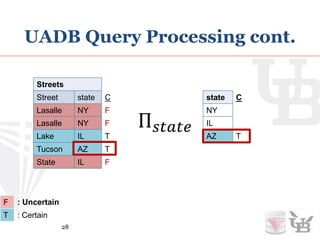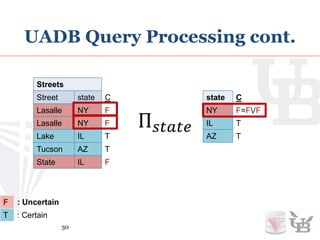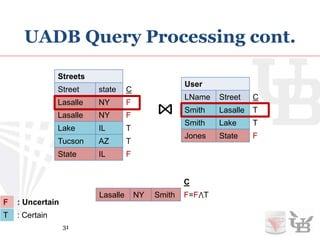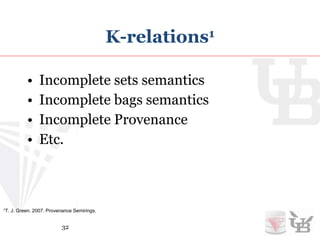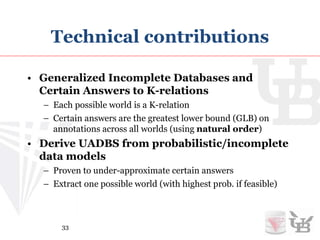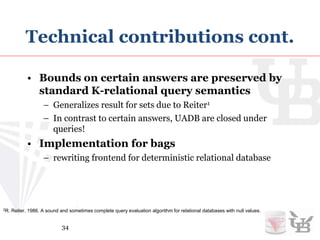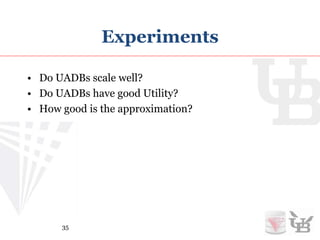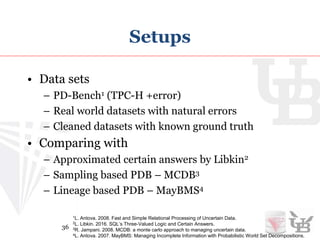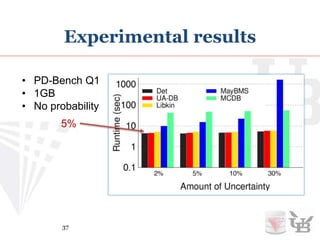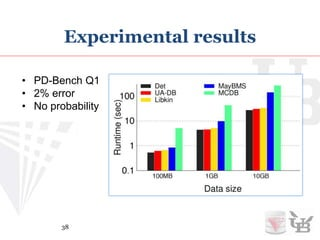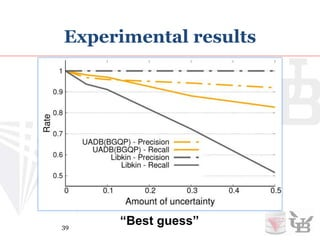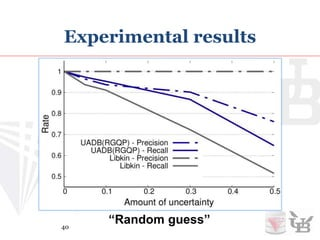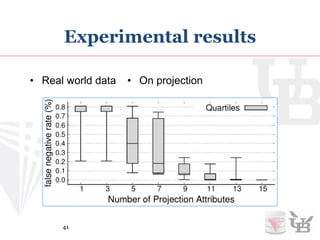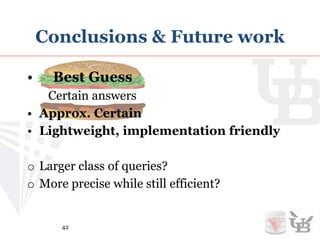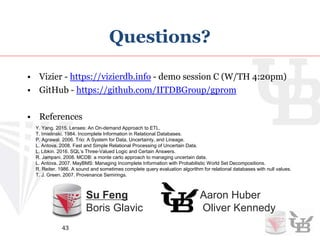1 of 43

### Editor's Notes

1. Uncertainty is everywhere.
2. Different sensors on same target may give you different readings.
3. Data cleaning may introduced uncertainty.
4. Information extraction like Searching for a street name may return multiple options.
5. For example. If we are unsure about which address is correct. We can record all possible options. In this case we do not know if state street is in IL, IN or CA. BTW this specific representation is called X-tuples.
6. And if we have a list of those options. Every row represents a tuple and every row with multiple options represents a decision. Each combination of the decisions for each row represents a possible instance and we call it possible world. For example choosing marked options will result the possible world on the right hand side.
7. In principal we can enumerate all possible worlds. For example these three possible worlds and so on. Then we get a naïve representation of incomplete database model. We can also assign probabilities to each possible worlds in which case we call it probabilistic database.
8. Of course we want to run queries over it.
9. Naively, We can do this by running the query over each possible worlds to get all possible outcomes. For example if we only have three possible worlds and we distinct project on state. Then we get these corresponding results for each possible world. If probabilities are assigned. We add probabilities when two or more worlds produce identical result. However this is completely non-practical since the number of possible world can be exponential to the number of decisions we have to make about the data. People come up several ways to deal with it more effectively.
10. Work on Full PDBs is hard. There are extensive works doing good job on compactly represent PDBs and query over them with relatively better performance. Most of them are either lineage based or sampling based. #p to compute actual probabilities. Polynomial probability approximation but those systems still need to track all or a large set of possible answers. Which still have performance bottleneck.
11. Here is a performance comparison between conventional query processing and the two types probabilistic databases just introduced. We can see that in the best case PQP is 3 times slower that conventional query processing and as uncertainty increases PQP can be up to 300 time slower in our test case.
12. So, PDBs are very expressive by tracking all possible answers and their probabilities. but doing so is a lot of work which make it very slow.
13. What If we do not have probabilities. And since possible answers can be very large, what if we only keep certain answers. Certain answers means the answers in common across all possible worlds. For example if we consider Id as one of the attribute, then those two tuples exist in all possible worlds so they are the certain answers. However, exact certain answers are still expensive to compute.
14. Thanks to the previous works we can compute approximated certain answers efficiently. And also the answers is a lower bound. subset of actual certain answers.
15. So, Approximated certain answers is efficient, but It does not track all possible answers as well as their probabilities. Also, since certain answers only give you a subset of the query result, how bad is this in practice?
16. In order to test that (how certain answers approach behaves). Recall that in reality, there is always a ground truth that is fundamentally correct. Of course the ground truth is unknown, but lets assume we know it. Certain answers are guaranteed to be a subset of the ground truth. However, It could be only a very conservative approximation of it.
17. So if we know the ground truth, we can measure the utility in terms of precision and recall of the certain answers from the ground truth versus the amount of uncertainty which shown on the graph. One sentence to describe the graph, certain answers will have high precision, low recall.
18. So, Certain answers lacking the utility by dropping all possible tuples. These are the principal approaches and they both gives you answers you can trust. Unfortunately, nobody uses them in practice.
19. In practice especially in many data cleaning tasks, people just make heuristic choices and ignore uncertainty afterwards. This is equivalent to picking one possible world. And we call it the best guess world. The down side of this approach is that we loss all information about uncertainty.
20. Although you can not trust any answers from the best guess world, you get the efficiency by only working on one world, get the utility by getting closer to the ground truth, There is no way you can get both efficiency and expressiveness. Since people want efficiency in practice, PDBs are out. For the other two approaches, one gives up utility, one gives up trustworthiness. Our approach – UADB achieves efficiency, trust-worthiness and utility. And for this, we have to give up expressiveness which means we are not tracking all possible answers.
21. The obvious solution for that is to combine approximated certain answers with best guess. We noticed that approximated certain answers are always a subset of the best guess world.
22. So we can use heuristics to find a best guess world and mark weather the tuple is an certain (blue tuples) or uncertain (red tuples) . Remember that the approximation of certain answers would be a subset of the actual certain answers. Every tuple we marked as certain is guaranteed to be certain. Every tuple we marked as uncertain may or may not be uncertain. But we are always bounding the actual certain answers between the under-approximation and the best guess. This works because certain answers is a subset of every possible world.
23. Uncertainty comes from different sources and lots of existing work has come out ways to modeling uncertainty. We do not want to re-invent the wheel. So we want to be able to translate existing models of uncertainty into UADB. In our paper we present translations from three common representations: tuple independent database, c-tables and x-tuples. Adding new models is easy.
24. After we have a UADB instance, query over UADB is light weight and easy to implement. Our system is query rewriting based so the rewritten query that can run on any conventional database systems like postgres, oracle, etc.
25. For using a relational database, we need a way to encode UADB as relations. We implement color labeling as an attribute at the end of the table. Where false means uncertain and true means certain.
26. We have a representation, how do we rewrite queries to operate over the representation? Let look at a query of projection over state. Here is the result on the best guess world. What is the correct annotation that preserves bounding?
27. For the last tuple AZ, it is clear that there is certainly one AZ tuple in the input, so the output must contain AZ tuple, so we label it as certain.
28. For the second tuple IL, there are two IL tuples in the input correspond to it where one of them is certain and one of them is not. Since one of the IL tuple in the input is certain, no mater the other uncertain IL tuple exist or not we will always have the IL tuple in the output. So we label it as certain.
29. For the first tuple NY. Both of the two input NY tuples are uncertain. So the output NY tuple can be missing when both of its uncertain inputs are missing. So we label it as uncertain.
30. For another example of cross join, if we only show casing the join of this two specific tuples. We can see that if we join a uncertain tuple with a certain tuple, the output tuple is uncertain since as long as one of the joining tuples is missing, the output tuple will not exist. Now, Many audiences may feel these examples looked very familiar. And it is! because we are using k-relations.
31. Which means our approach can works not only on uncertain sets but also uncertain bags, uncertain provenance, or many other semirings with querying semantics adaptable to k-relations.
32. For a summarized technical contributions, our approach..
33. We use both synthetic datasets which is TPC-H with randomly generated errors and also real world datasets.
34. When run our approach on synthetic dataset measuring runtime in log scale versus uncertainty percentage, we can see our approach have roughly 5% overhead comparing with conventional query processing. The low overhead maintained while the amount of uncertainty increase.
35. The low overhead also scales with data size.
36. In terms of utility, if we use a good case given by a good ML model i.e. spark ML, We can get precision over 90% and recall over 80%.
37. And if we do not have information or too expensive to get the best guess world. Here is the result if we randomly picking one world.
38. This is One out of the ten examples of the result showing misclassification of certain on real world data. It really depend on the data itself but in this case we get a worst case of 0.8% missclassified tuples.
39. By combining best guess with under approximation of certain answers we developed an uncertainty model that bounds actual certain answers, lightweight and easy to implement.
40. Our model also plays a role in Vizier system, so feel free to try Vizier demo too!